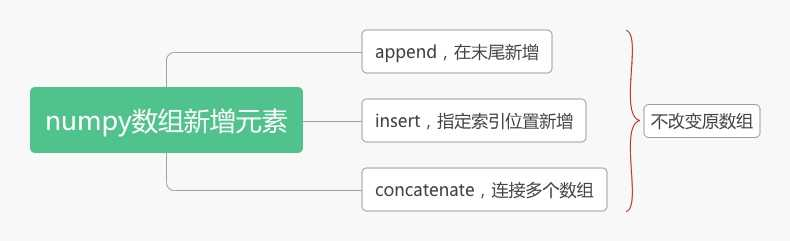# 第5讲，向numpy数组中新增元素1. apppend
2. insert
3. extend
4. 数组相加

### 1. append

``````import numpy as np

array = np.arange(10)
print(array)        # [0 1 2 3 4 5 6 7 8 9]

array = np.append(array, 11)
print(array)        # [ 0  1  2  3  4  5  6  7  8  9 11]
``````

append函数会返回一个新的ndarray，我将其重新赋值给array变量。

### 2. insert

``````import numpy as np

array = np.arange(10)
print(array)        # [0 1 2 3 4 5 6 7 8 9]

array = np.insert(array, 0, -1)
print(array)        # [-1  0  1  2  3  4  5  6  7  8  9]
``````

### 3. 列表的extend 和 numpy的concatenate

``````import numpy as np

array = np.arange(10)
print(array)        # [0 1 2 3 4 5 6 7 8 9]

array2 = np.array([11, 12])
array = np.concatenate((array, array2))
print(array)            # [ 0  1  2  3  4  5  6  7  8  9 11 12]
``````

concatenate函数可以将多个ndarray连接到一起返回一个新的数组

### 4. 数组相加

``````print([1, 2] + [3, 4])   # [1, 2, 3, 4]
``````

``````import numpy as np

array_1 = np.ones(3)
array_2 = np.ones(3)
print(array_1)      # [1. 1. 1.]
print(array_2)      # [1. 1. 1.]

array_3 = array_1 + array_2
print(array_3)      # [2. 2. 2.]
``````

``````import numpy as np

array_1 = np.ones(2)
array_2 = np.ones(3)
print(array_1)      # [1. 1. ]
print(array_2)      # [1. 1. 1.]

array_3 = array_1 + array_2
print(array_3)      # ValueError: operands could not be broadcast together with shapes (2,) (3,)
``````

array_1的shape与array_2的shape不同，因此不能做加法运算。

### 5. 总结

append，insert，concatenate都是numpy库的函数，不是numpy数组的方法。使用这些函数向ndarray中新增数据，并不能修改原数组，只是返回一个新的数组QQ交流群: 211426309